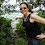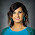## 12.24.2011

### Factoring ax^2 + bx + c

After reading Amy's post about airplane factoring, I decided to share the way I teach factoring.

I start out by teaching how to pull out the GCF, how to factor by grouping, then how to factor x^2 + bx + c using the diamond or the x-factor method. Those kind of have to be taught before this method. But it works.

I used the same problems she did so that you can compare the different methods.

The original problem

Multiply the first and last numbers. This answer, -12, is the top of the x.
The bottom of the x is the coefficient of x, in this case -1.
The sides of the x are two numbers that multiply to equal -12 and add to equal -1.

The students have a LOT of practice with the x-factor so they find that its -4 and 3.
Now they rewrite the problem, replacing the -x with a -4x + 3x.

Draw in our parentheses and now we have factoring by grouping, which students are also very familiar with.

Pull the GCF of each binomial out in front (in green).
Write what's left in parentheses (in pink).
Final answer: Write the common binomial first (in pink).
Write what's left behind. (in green).

Ta-da!

You've just been x-factored!

Another example for comparison.

1.This is what I do, but I love the X organizationand X factor name! Thanks for sharing!

iisanumber.blogspot.com

1.2.Thanks, I know I'm late to the game, but I love the x-factor organization!
@mrgjordan

3.It's probably one of my most favorite things!

4.Is there a mistake in the last picture? If I understood well there should be +4 in the right corner of the diamond. But really nice method thanks a lot

1.